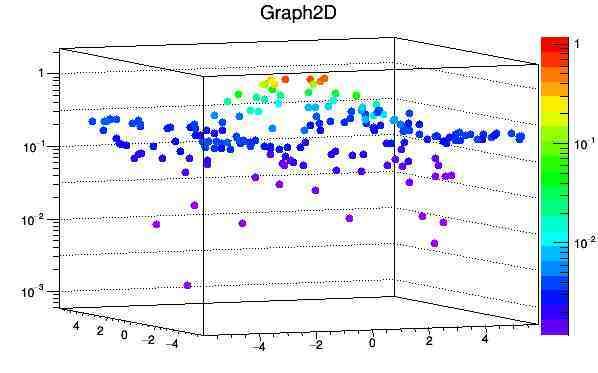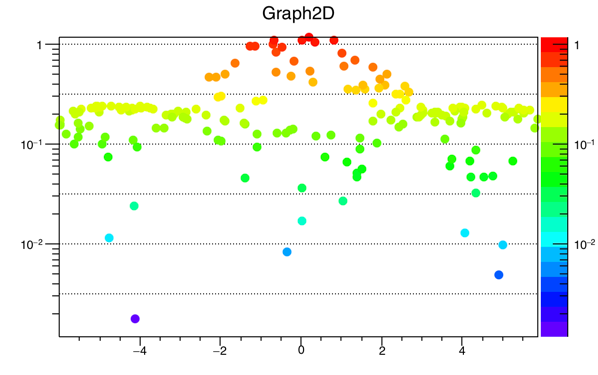# TGraph2D: logarithmic z-axis

Hi,

I’d like to plot a TGraph2D with a logarithmic z-axis. TPad::SetLogz() will set the z-axis to log-scale and adjust the TPaletteAxis, but the color palette does not translate to the graph. It works for TH2F though. You can see this from the following example, which is derived from the standard example in the TGraph2D documentation.

Any idea what is going on here and how to fix it?

Thanks,
Jochen

``````{
//logarithmic z-axis - color palette:
//it works for TH2F but not for TGraph2D

TCanvas *c = new TCanvas("c","Graph2D example",0,0,600,800);
c->Divide(1,2);
c_1->SetLogz();
c_2->SetLogz();

Double_t x, y, z, P = 6.;

Int_t np_g = 200;
Int_t np_h = 20000;
TGraph2D *dt = new TGraph2D();
TH2F *h = new TH2F("TH2F","TH2F",50,-6,6,50,-6,6);
TRandom *r = new TRandom();

for (Int_t N=0; N<np_g; N++) {
x = 2*P*(r->Rndm(N))-P;
y = 2*P*(r->Rndm(N))-P;
z = (sin(x)/x)*(sin(y)/y)+0.2;
dt->SetPoint(N,x,y,z);
}

for (Int_t N=0; N<np_h; N++) {
x = 2*P*(r->Rndm(N))-P;
y = 2*P*(r->Rndm(N))-P;
z = (sin(x)/x)*(sin(y)/y)+0.2;
h->Fill(x,y,z);
}

gStyle->SetPalette(1);
h->SetStats(0);
//h->GetZaxis()->SetRangeUser(1e-3,5);
//dt->GetZaxis()->SetRangeUser(1e-3,5);

c->cd(1);
dt->Draw("tri1z");
c->cd(2);
h->Draw("surf2z");
}``````

You should have an old version of ROOT. With 5.34 on Mac your macro gives me the attached picture.

Thanks, Olivier. I have version 5.26. Looks like time to upgrade…

Hi,

I have upgraded to the current ROOT version v5.34 as suggested. Unfortunately, I am running into a related problem now. The command TGraph2D::GetZaxis()->SetRangeUser(a,b) is ignored in the macro. Adjusting the axis “by hand” afterwards in the TPad works, but once again the color palette does not translate to the graph. Similarly, if I use TGraph2D::SetMinimum(a) and TGraph2D::SetMaximum(b) in the macro, the z-axis and the TPaletteAxis is adjusted, but it does not translate to the graph. See for macro and plot below.

The reason I want to do this is to have two TGraph2Ds back to back with the same z-axis range for better comparison.

Thanks,
Jochen

``````{
//logarithmic z-axis - color palette:
//it works for TH2F but not for TGraph2D

TCanvas *c = new TCanvas("c","Graph2D example",0,0,600,800);
c->Divide(1,2);
c_1->SetLogz();
c_2->SetLogz();

Double_t x, y, z, P = 6.;

Int_t np_g = 200;
Int_t np_h = 20000;
TGraph2D *dt = new TGraph2D();
TH2F *h = new TH2F("TH2F","TH2F",50,-6,6,50,-6,6);
TRandom *r = new TRandom();

for (Int_t N=0; N<np_g; N++) {
x = 2*P*(r->Rndm(N))-P;
y = 2*P*(r->Rndm(N))-P;
z = (sin(x)/x)*(sin(y)/y)+0.2;
dt->SetPoint(N,x,y,z);
}

for (Int_t N=0; N<np_h; N++) {
x = 2*P*(r->Rndm(N))-P;
y = 2*P*(r->Rndm(N))-P;
z = (sin(x)/x)*(sin(y)/y)+0.2;
h->Fill(x,y,z);
}

gStyle->SetPalette(1);
h->SetStats(0);
h->GetZaxis()->SetRangeUser(1e-3,1e3);
//dt->GetZaxis()->SetRangeUser(1e-3,1e3); //this command is ignored
dt->SetMaximum(1e3);
dt->SetMinimum(1e-3);

c->cd(1);
dt->Draw("tri1z");
c->cd(2);
h->Draw("surf2z");
}``````

I see what you mean. The TGraph2D plot uses the whole palette unlike the TH2 which use the correct range of colors. I am investigating.

This problem is now fixed in 534 and in the trunk.
With your macro, I now get the attached picture.

Thanks a lot Olivier for the excellent support! I am very impressed with the promptness of your replies and the fix. I managed to get my plot done with the trunk version obtained from git.

Jochen

That’s just my jobThanksHi, it seems that this problem comes back in Root 6.07/07.
I test these codes:

``````{
TCanvas *c = new TCanvas("c","Graph2D example",0,0,600,400);
Double_t x, y, z, P = 6.;
Int_t np = 200;
TGraph2D *dt = new TGraph2D();
TRandom *r = new TRandom();
for (Int_t N=0; N<np; N++) {
x = 2*P*(r->Rndm(N))-P;
y = 2*P*(r->Rndm(N))-P;
z = (sin(x)/x)*(sin(y)/y)+0.2;
dt->SetPoint(N,x,y,z);
}
gStyle->SetPalette(1);
dt->SetMarkerStyle(20);
dt->Draw("pcolz");
return c;
}``````

And get the result as the attached figure.
Could any one verify this and give me a solution?

Thanks,
HieuIt might be an other problem because you are not using the same plotting option. I will check.

Can you explain what you think is wrong in the plot you get … seems to me it looks fine …
I am surely missing something …

You can see there is a mismatch between the colors of points and the color’s z-values.
For example, in the 3D graph, the blue points correspond to z=210^(-1). However, on the color palette, the blue color corresponds to z=510^(-3).

If you plot this graph in linear scale of z. Such thing does not happen.

Both ROOT 5.34/36 and 6.06/04 behave the same way.
The graph is drawn with log-z but the color is still linear-z (improperly shown in the palette’s axis as being log, too).

I see. I hope that ROOT developers will consider this as a bug to be fixed soon.

You may open a bug report on JIRA in order to keep track of that problem.

I don’t have a CERN account to create a bug report in Jira. It seems that an external light weight account that I created doesn’t work.
Can any one of you help to create such bug report?
Thanks.

Now fixed in master:

``````{
TCanvas *c = new TCanvas("c","Graph2D example",0,0,600,400);
Double_t x, y, z, P = 6.;
Int_t np = 200;
TGraph2D *dt = new TGraph2D();
TRandom *r = new TRandom();
for (Int_t N=0; N<np; N++) {
x = 2*P*(r->Rndm(N))-P;
y = 2*P*(r->Rndm(N))-P;
z = (sin(x)/x)*(sin(y)/y)+0.2;
dt->SetPoint(N,x,y,z);
}
gStyle->SetPalette(1);